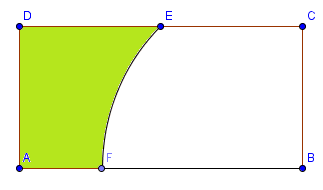# Area calculating part 3

Geometry Level 3$ABCD$ is a rectangle with sides $AB = 2$ and $BC = 1$. $EF$ is a circular arc with point $B$ as its centre. Also, point $E$ is a midpoint of the segment $CD$. If the green region can be represented as $\frac{1}{a}(b - \pi)$, where $a$ and $b$ are both positive integers, find the value of $a + b$

×

Problem Loading...

Note Loading...

Set Loading...